# KMP算法，看这篇就够了！

## 普通的模式匹配算法(BF算法)

#### 子串的定位操作通常称为模式匹配算法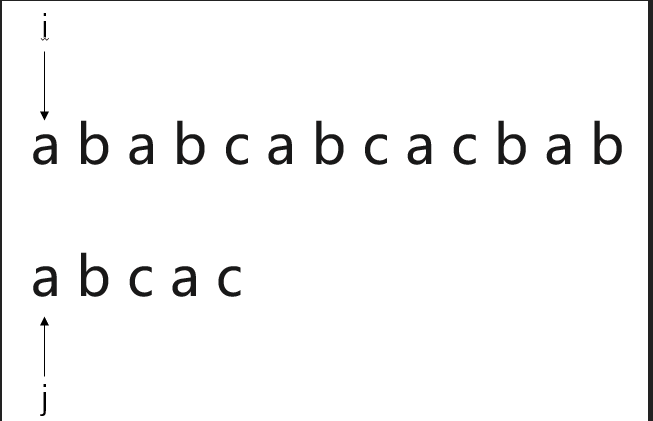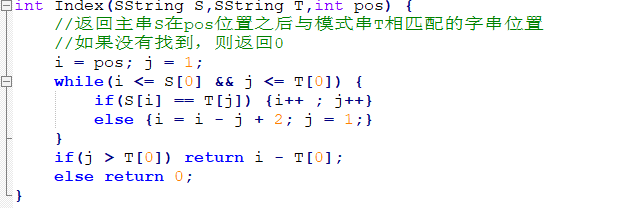BF算法确实实现了模式匹配的目的，但同时也有较大的缺陷

## 模式匹配改进算法(KMP算法)的引出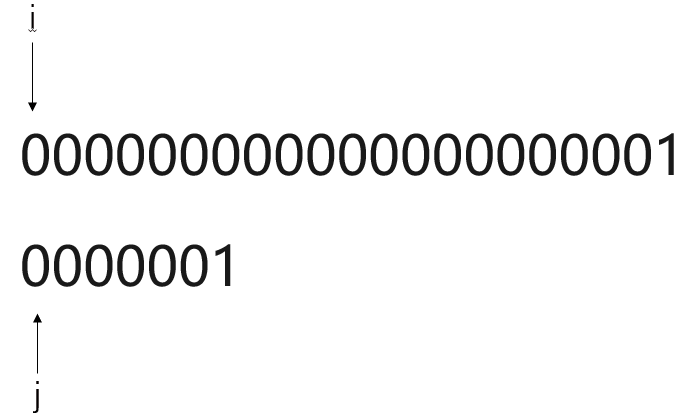O(m*n)

KMP算法

## KMP算法

#### KMP算法基本概念

KMP算法可以在时间复杂度为O(m+n)的时间数量级上完成模式匹配操作。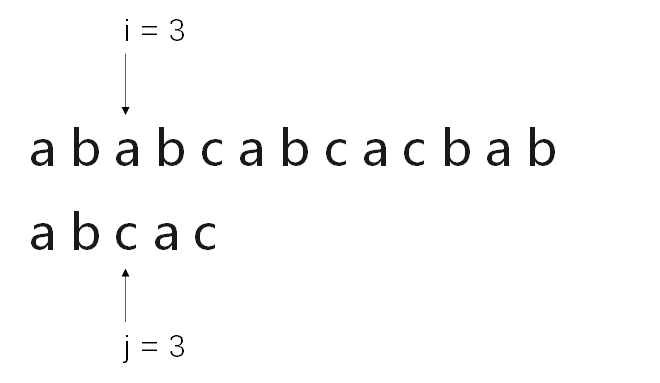(KMP算法比对过程1)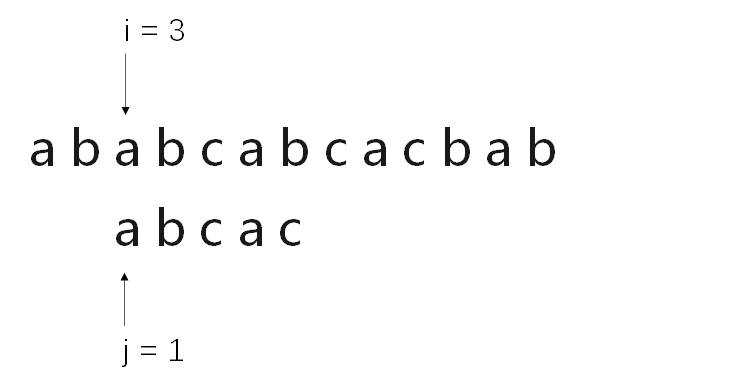(KMP算法比对过程2)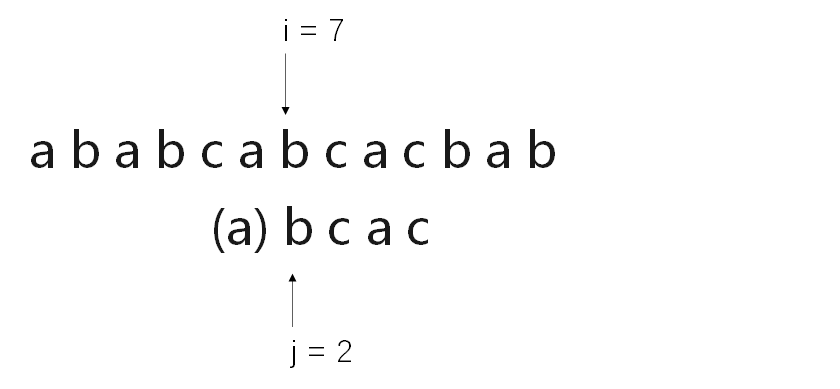(KMP算法比对过程3)

#### 滑动的条件

$p_1p_2...p_{k-1} = s_{i-k+1}s_{i-k+2}...s_{i-1}$

$p_{j-k+1}p_{j-k+2}...p_{j-1} = s_{i-k+1}s_{i-k+2}...s_{i-1}$

$p_1p_2...p_{k-1} = p_{j-k+1}p_{j-k+2}...p_{j-1}$

#### 滑动的模式

next[j] 条件 结果
0 当j等于1 将i向后移动一位
k 取Kmax，1<k<j 且 满足条件③ 将模式串的第k位与当前i对齐
1 其他情况 将模式串的第1位与当前i对齐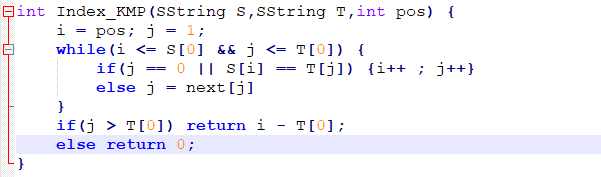#### 滑动距离K的求解

$p_1p_2...p_{k-1} = p_{j-k+1}p_{j-k+2}...p_{j-1}$

• 可知next[j]仅与模式串有关而与主串无关

$p_k = p_j$

$p_k ≠ p_j$

next[j+1] = next[j] + 1;

1. 设K' = next[k],当P[K'] = P[j] 时，有
next[j+1] = next[k] + 1;
2. 如果一直移动K'到j = 1时，还不能找到对应的P[K'] = P[j],那么直接有
next[j+1] = 1;

• k，j，k'，j+1分别代表串中的哪些位置

1. j+1就是你需要求k值的模式串位置
2. j就是当前需要求出K值的字符的前一个字符
3. k-1就是“已匹配”的前缀的最后一个字符
4. k就是“已匹配”的前缀的最后一个字符的后一个字符

• 前缀一定要包含第1个字符
• 后缀一定要包含希望求得K的字符的前一个字符• 重点来了
让我们抛出一个例子，来理解next[j]的求法
假设我们已知j=1，2，3，4的next[j]值分别为：0，1，1，2
由于k-1和j-1已经”匹配“，则满足前置匹配条件，我们来比较pk和pj的内容，
pk = b，pj = a；
可见它们并不相等，因此k指向的b会甩锅给“next[k]”字符，而next[k] = 1，也就是串的第一个字符‘a'
第一个字符‘a'成功与第j个字符‘a'相匹配，因此依照我们定义的的pk ≠ pj的第一种情况可以得到
next[j+1] = next[k] + 1 = 2;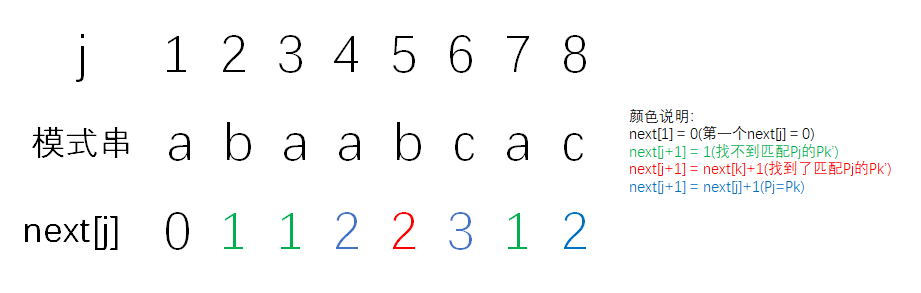• k，j相等，直接加1
• k，j不等，层层甩锅
• 甩锅失败，直接赋1
• 甩锅成功，老板加1

1. pk = pj：next[j+1] = next[j] + 1;
2. pk ≠ pj: 甩锅
3. p[k'] = pj:next[j+1] = next[k] + 1;
4. p[k'] ≠ pj:next[j+1] = 1 or 继续向next[k']甩锅。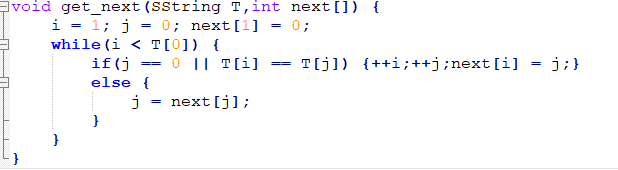#### KMP算法的改进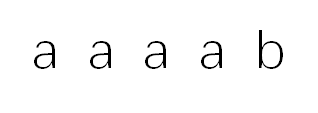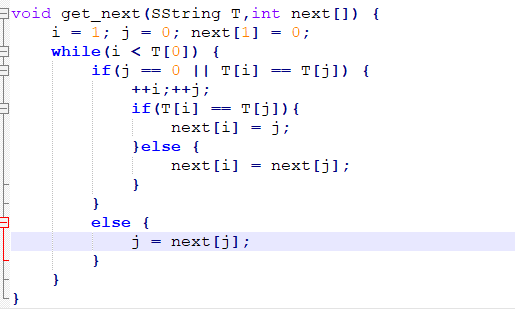posted @ 2021-09-13 22:40  Tayoou  阅读(242)  评论(0编辑  收藏  举报